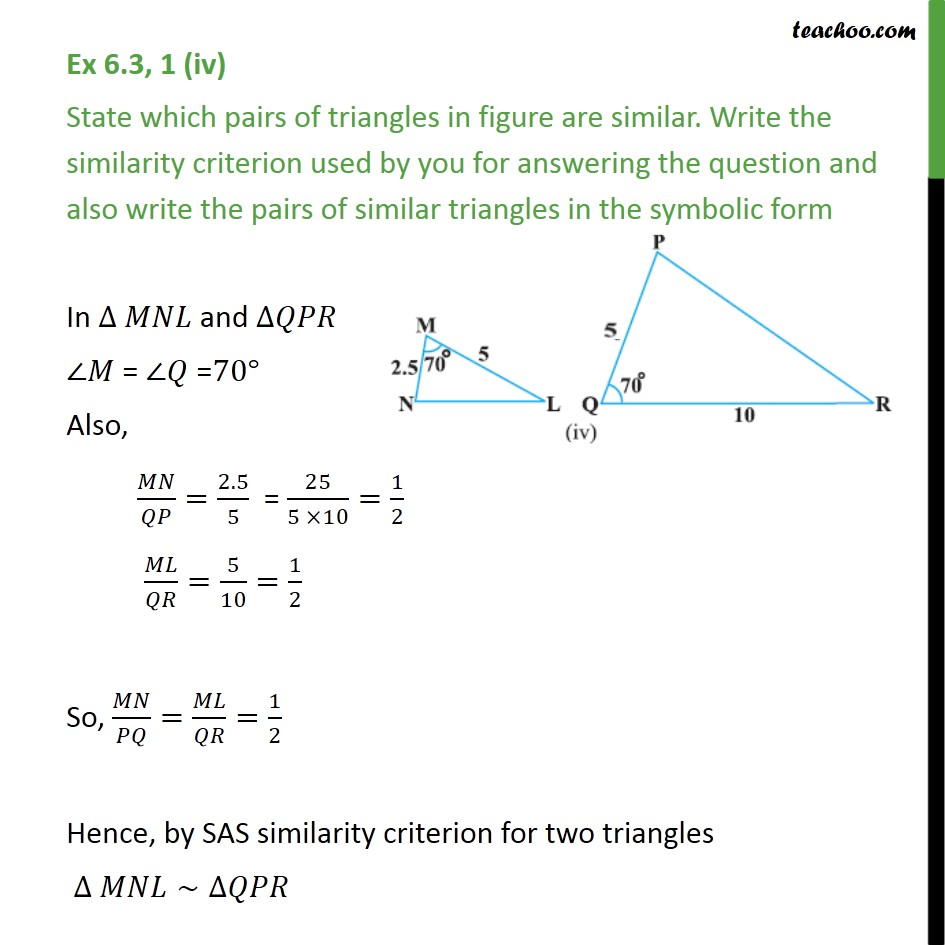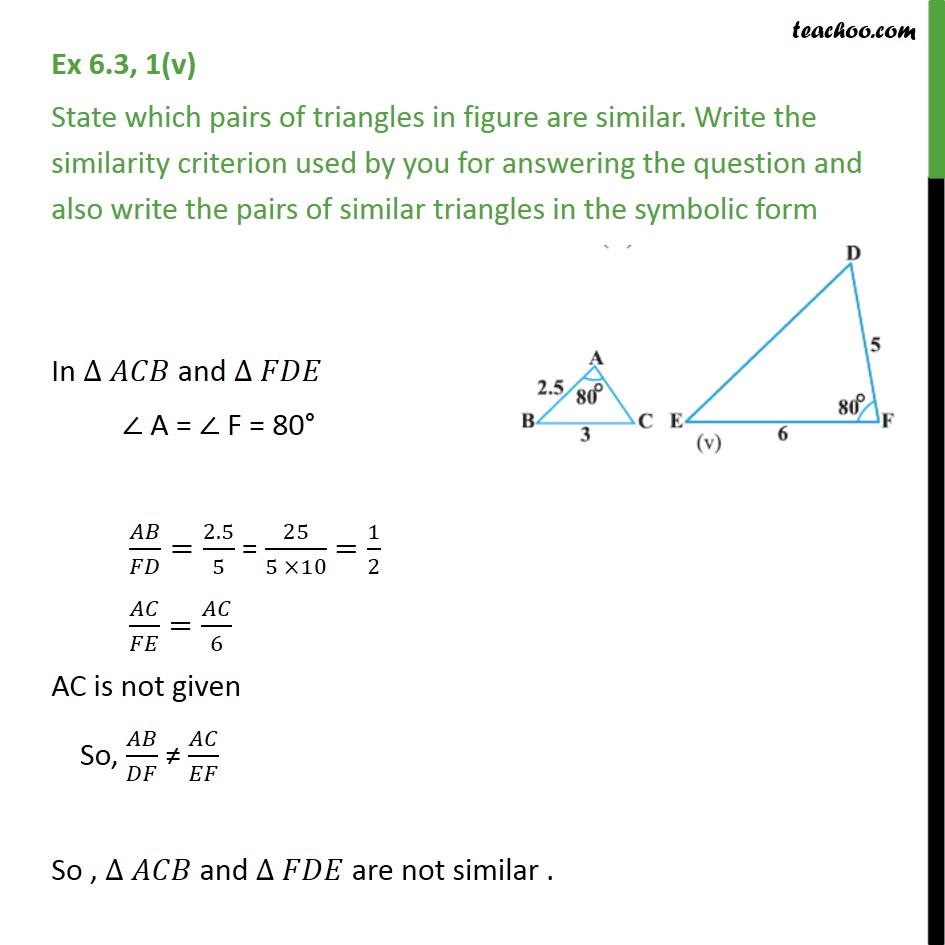1. Chapter 6 Class 10 Triangles
2. Concept wise
3. SAS Similarity

Transcript

Ex 6.3, 1 (iv) State which pairs of triangles in figure are similar. Write the similarity criterion used by you for answering the question and also write the pairs of similar triangles in the symbolic form In ∆ 𝑀𝑁𝐿 and ∆𝑄𝑃𝑅 ∠𝑀 = ∠𝑄 =70° Also, 𝑀𝑁/𝑄𝑃=2.5/5 = 25/(5 ×10)=1/2 𝑀𝐿/𝑄𝑅=5/10=1/2 So, 𝑀𝑁/𝑃𝑄=𝑀𝐿/𝑄𝑅=1/2 Hence, by SAS similarity criterion for two triangles ∆ 𝑀𝑁𝐿∼∆𝑄𝑃𝑅 Ex 6.3, 1(v) State which pairs of triangles in figure are similar. Write the similarity criterion used by you for answering the question and also write the pairs of similar triangles in the symbolic form In ∆ 𝐴𝐶𝐵 and ∆ 𝐹𝐷𝐸 ∠ A = ∠ F = 80° 𝐴𝐵/𝐹𝐷=2.5/5 = 25/(5 ×10) =1/2 𝐴𝐶/𝐹𝐸=𝐴𝐶/6 AC is not given So, 𝐴𝐵/𝐷𝐹 ≠ 𝐴𝐶/𝐸𝐹 So , ∆ 𝐴𝐶𝐵 and ∆ 𝐹𝐷𝐸 are not similar .

SAS Similarity

About the AuthorDavneet Singh
Davneet Singh is a graduate from Indian Institute of Technology, Kanpur. He has been teaching from the past 9 years. He provides courses for Maths and Science at Teachoo.